# High School Chemistry : Help with Reaction Order

## Example Questions

### Example Question #1 : Help With Reaction Order

Consider the following equation: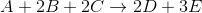The initial rate of the reaction was recorded for varying reactant concentrations and was recorded in a table: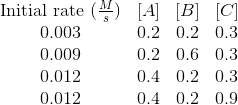Based on these experiments, what is the rate law for this reaction?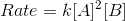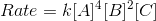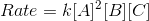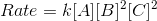Explanation:

The balanced reaction does not tell you anything about the rate law of a reaction. The rate law must be determined through experiment. The basic format of our rate law will be: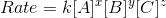In order to see how the initial concentration of a reactant affects the initial rate of the reaction, we need to find two trials where two reactants are kept constant and only one is changed. By seeing how the initial reaction rate changes, we can determine the reaction order for each particular reactant and fill in the exponent values.

When the concentration of A is doubled, the initial reaction rate is quadrupled. This can be seen by comparing trials 1 and 3. As a result, the reaction is second order with respect to A.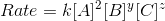When the concentration of B is tripled, the initial reaction rate is tripled. This can been seen by comparing trials 1 and 2. As a result, the reaction is first order with respect to B.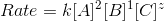Finally, the initial reaction rate will not change regardless of the concentration change of C. This can be seen by comparing trials 3 and 4. This makes the reaction zero order with respect to C.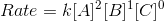### All High School Chemistry Resources